# planar graph

## Obstacle number of planar graphs ★

Author(s): Alpert; Koch; Laison

Does there exist a planar graph with obstacle number greater than 1? Is there somesuch that every planar graph has obstacle number at most?

## Finding k-edge-outerplanar graph embeddings ★★

Author(s): Bentz

Conjecture   It has been shown that a-outerplanar embedding for whichis minimal can be found in polynomial time. Does a similar result hold for-edge-outerplanar graphs?

Keywords: planar graph; polynomial algorithm

## Approximation ratio for k-outerplanar graphs ★★

Author(s): Bentz

Conjecture   Is the approximation ratio for the Maximum Edge Disjoint Paths (MaxEDP) or the Maximum Integer Multiflow problem (MaxIMF) bounded by a constant in-outerplanar graphs or tree-width graphs?

## Approximation Ratio for Maximum Edge Disjoint Paths problem ★★

Author(s): Bentz

Conjecture   Can the approximation ratiobe improved for the Maximum Edge Disjoint Paths problem (MaxEDP) in planar graphs or can an inapproximability result stronger than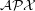-hardness?

## Domination in plane triangulations ★★

Author(s): Matheson; Tarjan

Conjecture   Every sufficiently large plane triangulationhas a dominating set of size.

Keywords: coloring; domination; multigrid; planar graph; triangulation

## 5-coloring graphs with small crossing & clique numbers ★★

Author(s): Oporowski; Zhao

For a graph, we let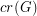denote the crossing number of, and we letdenote the size of the largest complete subgraph of.

Question   Does every graphwithand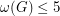have a 5-coloring?

Keywords: coloring; crossing number; planar graph

## Jones' conjecture ★★

Author(s): Kloks; Lee; Liu

For a graph, let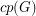denote the cardinality of a maximum cycle packing (collection of vertex disjoint cycles) and let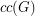denote the cardinality of a minimum feedback vertex set (set of verticesso thatis acyclic).

Conjecture   For every planar graph,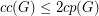.

Keywords: cycle packing; feedback vertex set; planar graph

## Oriented chromatic number of planar graphs ★★

Author(s):

An oriented colouring of an oriented graph is assignmentof colours to the vertices such that no two arcs receive ordered pairs of coloursand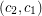. It is equivalent to a homomorphism of the digraph onto some tournament of order.

Problem   What is the maximal possible oriented chromatic number of an oriented planar graph?

Keywords: oriented coloring; oriented graph; planar graph

## Mapping planar graphs to odd cycles ★★★

Author(s): Jaeger

Conjecture   Every planar graph of girth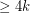has a homomorphism to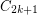.

Keywords: girth; homomorphism; planar graph

## Circular coloring triangle-free subcubic planar graphs ★★

Author(s): Ghebleh; Zhu

Problem   Does every triangle-free planar graph of maximum degree three have circular chromatic number at most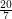?

Keywords: circular coloring; planar graph; triangle free